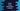# Introduction to Kotlin string interpolation with examples## Kotlin string interpolation:

Unlike Java, we have different ways to concatenate strings in Kotlin. The string interpolation in Kotlin allows us to include expressions or variables directly in the string template. We don’t have to use the plus symbol, + to concatenate strings.

Let’s take a look at the below example:

``````fun main() {
val num = 123
val w = "world"
val str = "Hello" + num + w + "!!"
print(str)
}``````

Here, we are concatenating four strings to create the final string str. We are using + to concatenate these strings.

If we use string interpolation for the above example, it will look as like below:

``````fun main() {
val num = 123
val w = "world"
val str = "Hello\$num\$w!!"
print(str)
}``````

As you can see here, \$ is used to interpolate the variables num and w. We can also use it with expressions.

Let me show you a couple of examples to understand how string interpolation works:

### Example 1: String interpolation with different types of variables:

Let’s take a look at the below example:

``````data class Student(val name: String, val age: Int)

fun main() {
val s = Student("Alex", 20)

println("The name of the student is \${s.name} and age is \${s.age}. The length of the name is \${s.name.length}")
}``````

Here, we created a data class Student and one object of this class s. This class has two properties, name and age. The last print statement is printing the name, age and length of the name.

It will print:

``The name of the student is Alex and age is 20. The length of the name is 4``

### Example 2: String interpolation example with functions:

We can also use string interpolation with functions i.e. it will execute the functions and include the return value to the final string.

``````fun divide(first: Int, second: Int): Int {
return first / second
}

fun multiply(first: Int, second: Int): Int {
return first * second
}

fun main() {
val firstNumber = 20
val secondNumber = 5

println(
"Given numbers: \$firstNumber, \$secondNumber.\n\$firstNumber/\$secondNumber = \${
divide(
firstNumber,
secondNumber
)
}\n\$firstNumber * \$secondNumber = \${multiply(firstNumber, secondNumber)}"
)

}``````

In this example,

• divide is a function to find the division of two numbers and multiply is a function to find the multiplication of two numbers. Both of these functions take two integers as the parameters and return another integer value.
• In the main function, we are creating a final string with string interpolation. It calls the divide and multiply functions and appends the result to the result string.